# ICSE Solutions for Chapter 19 Construction Class 10 Mathematics

Question 1: Draw a circle of radius 3 cm. Mark a point P at a distance of 5 cm from the centre of the circle drawn. Draw two tangents PA and PB to the given circle and measure the length of each tangent.

Solution 1:Steps Of Construction:
(i) Draw a circle with centre and radius 3 cm.
(ii) From O, take a point P such that OP = 5 cm
(iii) Draw a bisector of OP which intersects OP at M.
(iv) With centre M, and radius OM, draw a circle which intersects the given circle at A and B.
(v) Join AP and BP.
AP and BP are the required tangents.
On measuring AP = BP = 4 cm

Question 2: Draw a circle of diameter of 9 cm. Mark a point at a distance of 7.5 cm from the centre of the circle. Draw tangents to the given circle from this exterior point. Measure the length of each tangent.

Solution 2: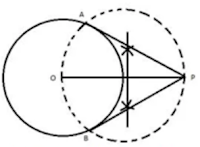(i) Draw a circle of diameter 9 cm, taking O as the centre.
(ii) Mark a point P outside the circle, such that PO = 7.5 cm.
(iii) Taking OP as the diameter, draw a circle such that it cuts the earlier circle at A and B.
(iv) Join PA and PB.

Question 3: Draw a circle of radius 5 cm. Draw two tangents to this circle so that the angle between the tangents is 45°.

Solution 3: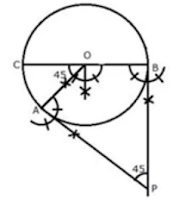Steps of Construction:
(i) Draw a circle with centre O and radius BC = 5 cm
(ii) Draw arcs making an angle of 180°- 45° = 135° at O such that ∠AOB = 135˚
(iii) AT A and B draw two rays making an angle of 90° at each point which meet each other at point outside the circle.
iv) AP and BP are the required tangents which make an angle of 45° with each other at P.

Question 4: Draw a circle of radius 4.5 cm. Draw two tangents to this circle so that the angle between the tangents is 60°

Solution 4:Steps of Construction:
(i) Draw a circle with centre and radius BC = 4.5 cm
(ii) Draw arcs making an angle of 180° - 60° = 120° at O such that ∠AOB = 120°
(iii) AT A and B, draw two rays making an angle of 90° at each point which meet each other at point P, outside the circle.
(iv) AP and BP are the required tangents which make an angle of 60° with each other at P.

Question 5: Using ruler and compasses only, draw an equilateral triangle of side 4.5 cm and draw its circumscribed circle. Measure the radius of the circle.

Solution 5:Steps of construction:
(i) Draw a line segment BC = 4.5 cm
(ii) With centers B and C. draw two arcs of radius 4.5 cm which intersect each other at A.
(iii) Join AC and AB.
(iv) Draw perpendicular bisectors of AC and BC intersecting each other at O.
(v) With centre O, and radius OA or OB or OC draw a circle which will pass through A, B and C.
This is the required circumcircle of triangle ABC.
On measuring the radius OA = 2.6 cm

Question 6: Using ruler and compasses only.
(i) Construct triangle ABC, having given BC = 7 cm, AB - AC = 1 cm and ∠ABC = 45˚
(ii) Inscribe a circle in the ΔABC constructed in (i) above. Measure its radius.

Solution 6:Steps of Construction:
(i) Construction of triangle:
(a) Draw a line segment BC = 7 cm
(b) At B, draw a ray BX making an angle of 45° and cut off BE = AB - AC = 1 cm
(c) Join EC and draw the perpendicular bisector of EC intersecting BX at A.
(d) Join AC. ΔABC is the required triangle

(ii) Construction of incircle:
(e) Draw angle bisectors of ∠ABC and ∠ACB intersecting each other at O.
(f) From O. draw perpendiculars OL to BC.
(g) O as centre and OL as radius draw circle which touches the sides of the ΔABC. This is the required in-circle of ΔABC.
On measuring, radius OL = 1.8 cm

Question 7: Using ruler and compasses only, draw an equilateral triangle of side 5 cm. Draw its inscribed circle. Measure the radius of the circle.

Solution 7: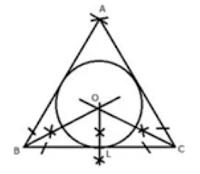Steps of Construction:
(i) Draw a line segment BC = 5 cm
(ii) With centers B and C, draw two arcs of 5 cm radius each which intersect each other at A.
(iii) Join AB and AC.
(iv) Draw angle bisectors of ∠B and ∠C intersecting each other at O.
(v) From O, draw OL ⊥ BC
(vi) Now with centre and radius OL, draw a circle which will touch the sides of AABC
On measuring, OL = 1.4 cm

Question 8: Using ruler and compasses only,
(i) Construct a triangle ABC with the following data:
Base AB = 6 cm, BC = 6.2 cm and ∠CAB = 60°
(ii) In the same diagram, draw a circle which passes through the points A, B and C and mark its centre as O.
(iii) Draw a perpendicular from O to AB which meets AB in D.
(iv) Prove that AD = BD

Solution 8: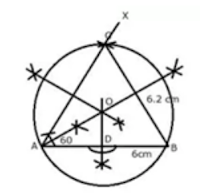Steps of construction:
(i) Draw a line segment AB = 6 cm
(ii) At A, draw a ray making an angle of 60° with BC.
(iii) With Bas centre and radius = 6.2 cm draw an arc which intersects AX ray at C. iv) Join BC.
ΔABC is the required triangle.
(v) Draw the perpendicular bisectors of AB and AC intersecting each other at O.
(vi) With centre O, and radius as OA or OB or OC, draw a circle which will pass through A, B and C.
(vii) From O draw OD ⊥ AB

Proof: In right ΔOAD and ΔOBD
OA = OB (radii of same circle)
Side OD = OD (common)

Question 9: Using ruler and compasses only construct a triangle ABC in which BC = 4 cm, ∠ACB = 45˚ and perpendicular from A on BC is 2.5 cm. Draw a circle circumscribing the triangle ABC.

Solution 9: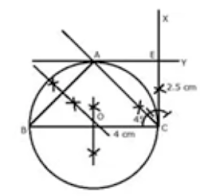Steps of Construction:
(i) Draw a line segment BC = 4 cm.
(ii) At C, draw a perpendicular line CX and from it, cut off CE = 2.5 cm.
(iii) From E, draw another perpendicular line EY.
(iv) From C, draw a ray making an angle of 45° with CB, which intersects EY at A.
(v) Join AB. ΔABC is the required triangle.
(vi) Draw perpendicular bisectors of sides AB and BC intersecting each other at O.
(vii) With centre O, and radius OB, draw a circle which will pass through A, B and C.
Measuring the radius OB = OC = OA = 2 cm

Question 10: Perpendicular bisectors of the sides AB and AC of a triangle ABC meet at O.
(i)What do you call the point O?
(ii) What is the relation between the distances OA, OB and OC?
(iii) Does the perpendicular bisector of BC pass through O?

Solution 10: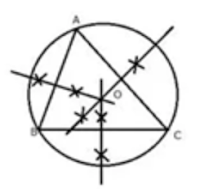(i) O is called the circumcentre of circumcircle of ΔABC.
(ii) OA, OB and OC are the radii of the circumcircle.
(iii) Yes, the perpendicular bisector of BC will pass through O.